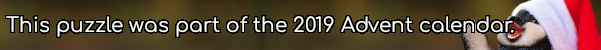mscroggs.co.uk
mscroggs.co.uksubscribeIn bases 3 to 9, the number 112 is: $$11011_3$$, $$1300_4$$, $$422_5$$, $$304_6$$, $$220_7$$, $$160_8$$, and $$134_9$$. In bases 3, 4, 6, 8 and 9, these representations contain no digit 2.